Want to share your content on R-bloggers? click here if you have a blog, or here if you don't.

To explain the “optimal transport” problem, we usually start with Gaspard Monge’s “Mémoire sur la théorie des déblais et des remblais“, where the the problem of transporting a given distribution of matter (a pile of sand for instance) into another (an excavation for instance). This problem is usually formulated using distributions, and we seek the “optimal” transport from one distribution to the other one. The formulation, in the context of distributions has been formulated in the 40’s by Leonid Kantorovich, e.g. from the distribution on the left to the distribution on the right.Consider now the context of finite sets of points. We want to transport mass from points to points . It is a complicated combinatorial problem. For 4 points, there are only 24 possible transfer to consider, but it exceeds 20 billions with 15 points (on each side). For instance, the following one is usually seen as inefficient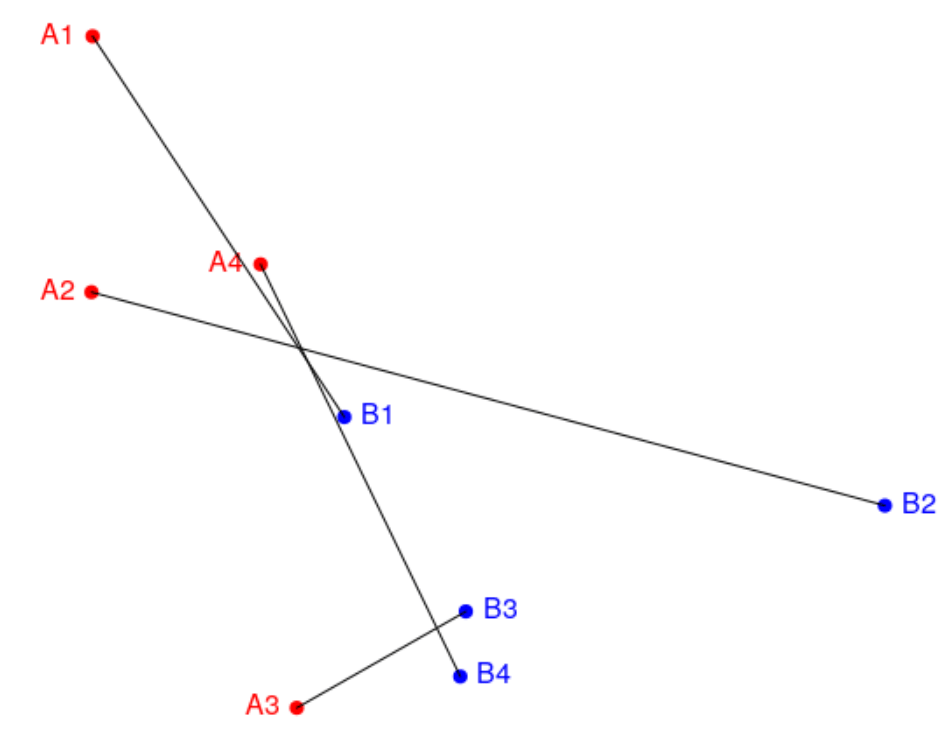while the following is usually seen as much better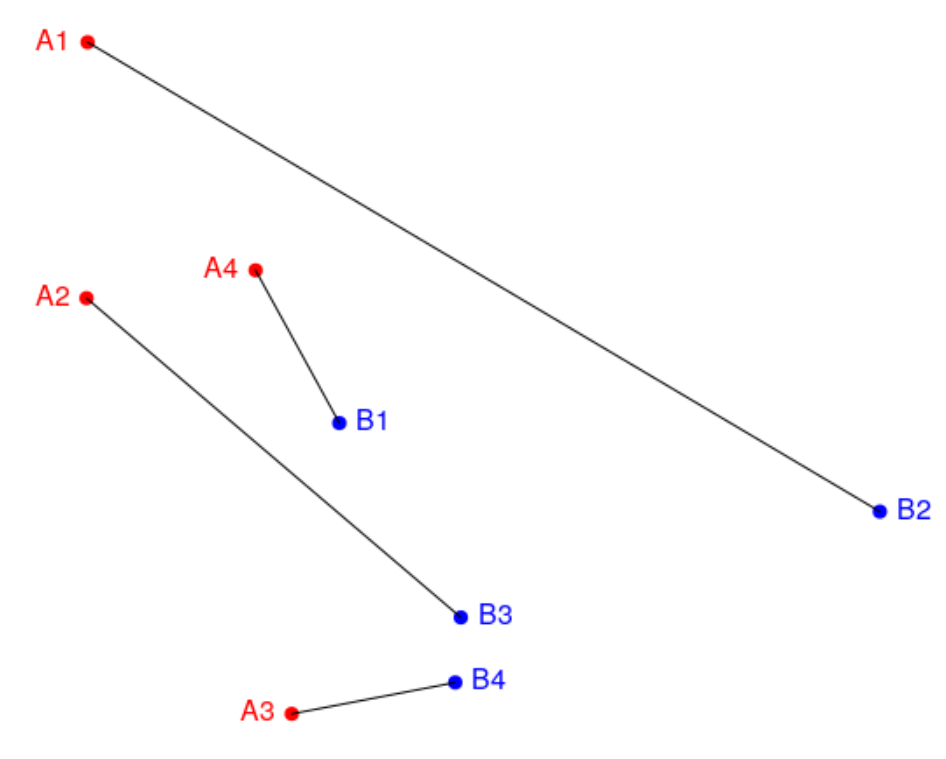Of course, it depends on the cost of the transport, which depends on the distance between the origin and the destination. That cost is usually either linear or quadratic.

There are many application of optimal transport in economics, see eg Alfred’s book Optimal Transport Methods in Economics. And there are also applications in statistics, that what I’ve seen while I was discussing with Pierre while I was in Boston, in June. For instance if we want to test whether some sample were drawn from the same distribution,

```set.seed(13) npoints <- 25 mu1 <- c(1,1) mu2 <- c(0,2) Sigma1 <- diag(1, 2, 2) Sigma2 <- diag(1, 2, 2) Sigma2[2,1] <- Sigma2[1,2] <- -0.5 Sigma1 <- 0.4 * Sigma1 Sigma2 <- 0.4 *Sigma2 library(mnormt) X1 <- rmnorm(npoints, mean = mu1, Sigma1) X2 <- rmnorm(npoints, mean = mu2, Sigma2) plot(X1[,1], X1[,2], ,col="blue") points(X2[,1], X2[,2], col = "red")```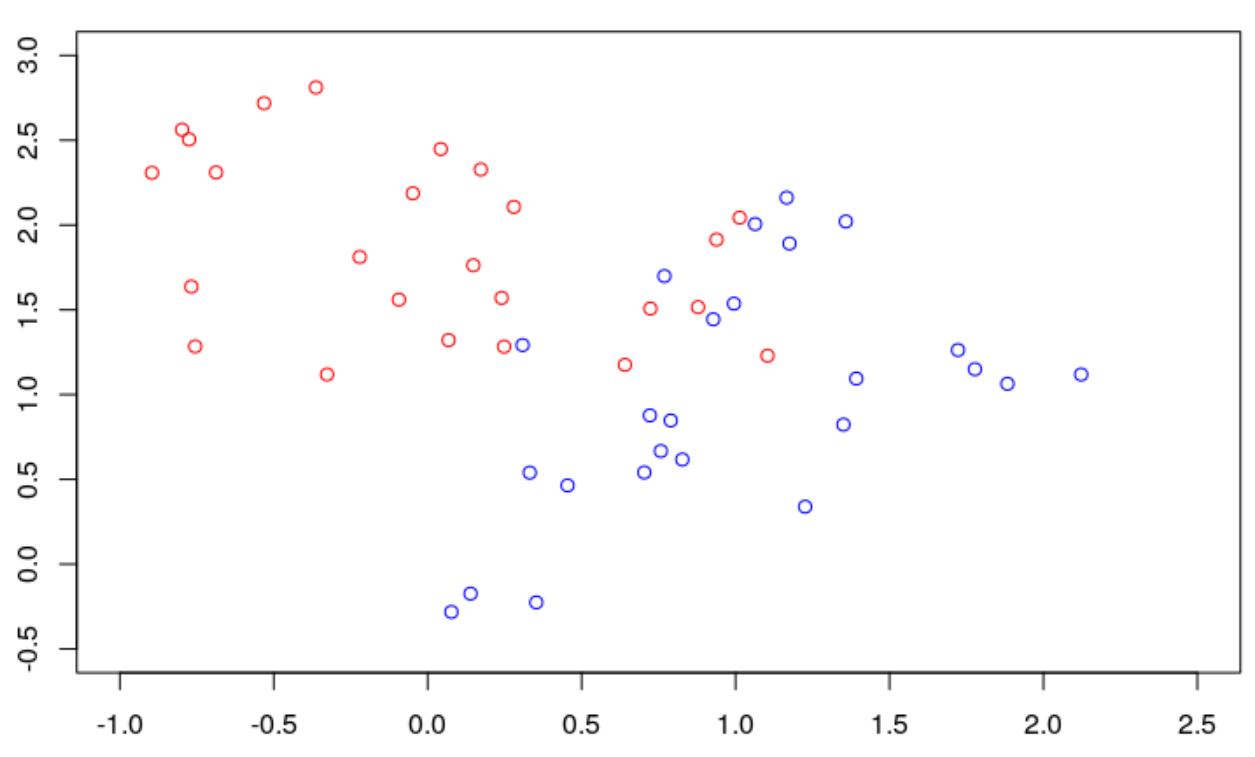Here we use a parametric model to generate our sample (as always), and we might think of a parametric test (testing whether mean and variance parameters of the two distributions are equal).or we might prefer a nonparametric test. The idea Pierre mentioned was based on optimal transport. Consider some quadratic loss

```ground_p <- 2 p <- 1 w1 <- rep(1/npoints, npoints) w2 <- rep(1/npoints, npoints) C <- cost_matrix_Lp(t(X1), t(X2), ground_p) library(transport) a <- transport(w1, w2, costm = C^p, method = "shortsimplex")```

then it is possible to match points in the two samples

```nonzero <- which(a\$mass != 0) from_indices <- a\$from[nonzero] to_indices <- a\$to[nonzero] for (i in from_indices){ segments(X1[from_indices[i],1], X1[from_indices[i],2], X2[to_indices[i], 1], X2[to_indices[i],2]) }```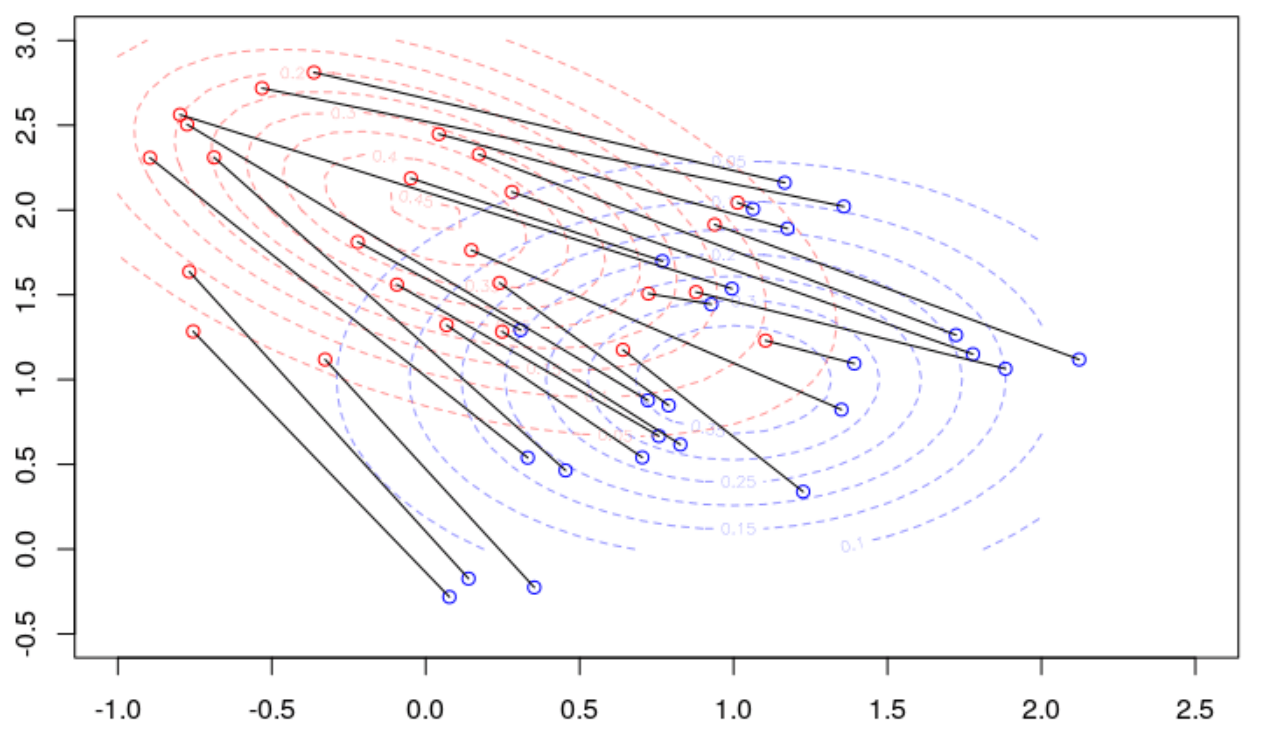Here we can observe two things. The total cost can be seen as rather large

```> cost=function(a,X1,X2){ nonzero <- which(a\$mass != 0) naa=a[nonzero,] d=function(i) (X1[naa\$from[i],1]-X2[naa\$to[i],1])^2+(X1[naa\$from[i],2]-X2[naa\$to[i],2])^2 sum(Vectorize(d)(1:npoints)) } > cost(a,X1,X2)  9.372472```

and the angle of the transport direction is alway in the same direction (more or less)

```> angle=function(a,X1,X2){ nonzero <- which(a\$mass != 0) naa=a[nonzero,] d=function(i) (X1[naa\$from[i],2]-X2[naa\$to[i],2])/(X1[naa\$from[i],1]-X2[naa\$to[i],1]) atan(Vectorize(d)(1:npoints)) } > mean(angle(a,X1,X2))  -0.3266797```

> library(plotrix)
> ag=(angle(a,X1,X2)/pi)*180
> ag[ag<0]=ag[ag<0]+360
> dag=hist(ag,breaks=seq(0,361,by=1)-.5)
> polar.plot(dag\$counts,seq(0,360,by=1),main=”Test Polar Plot”,lwd=3,line.col=4)

(actually, the following plot has been obtain by generating a thousand of sample of size 25)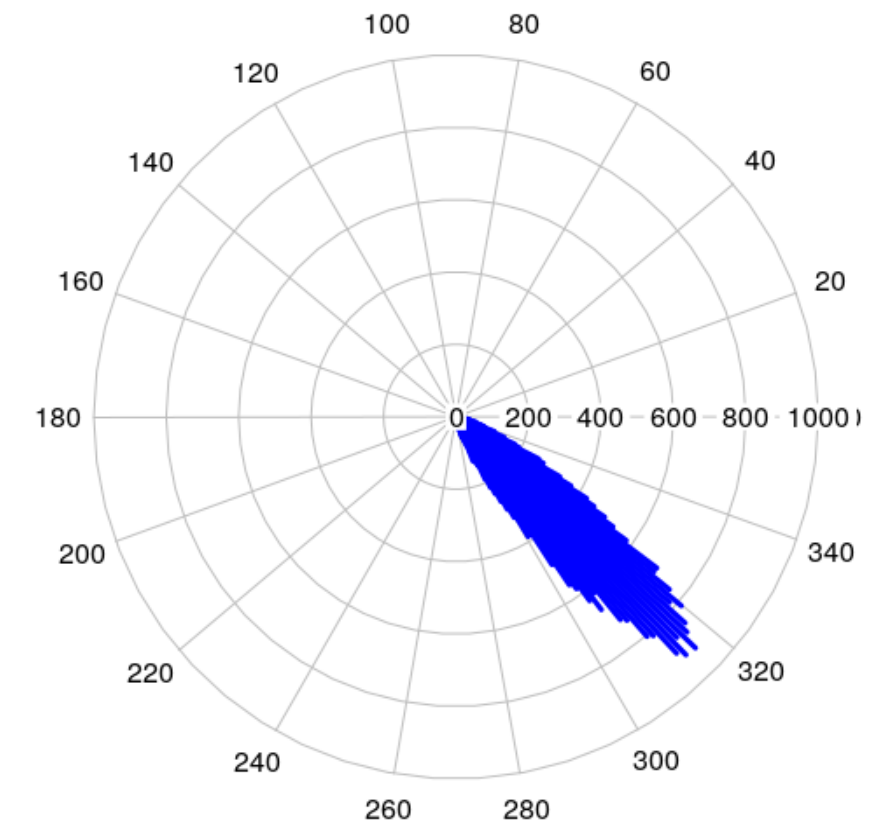In order to have a decent test, we need to see what happens under the null assumption (when drawing samples from the same distribution), see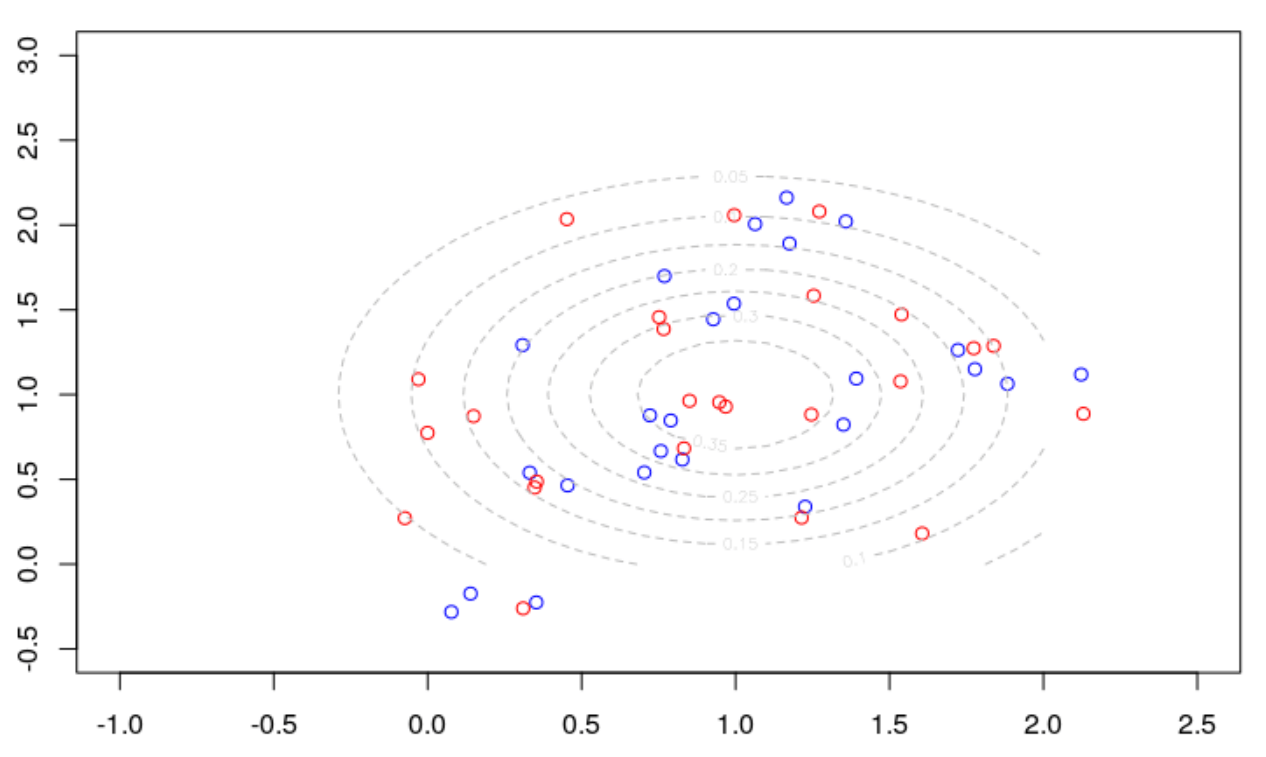Here is the optimal matching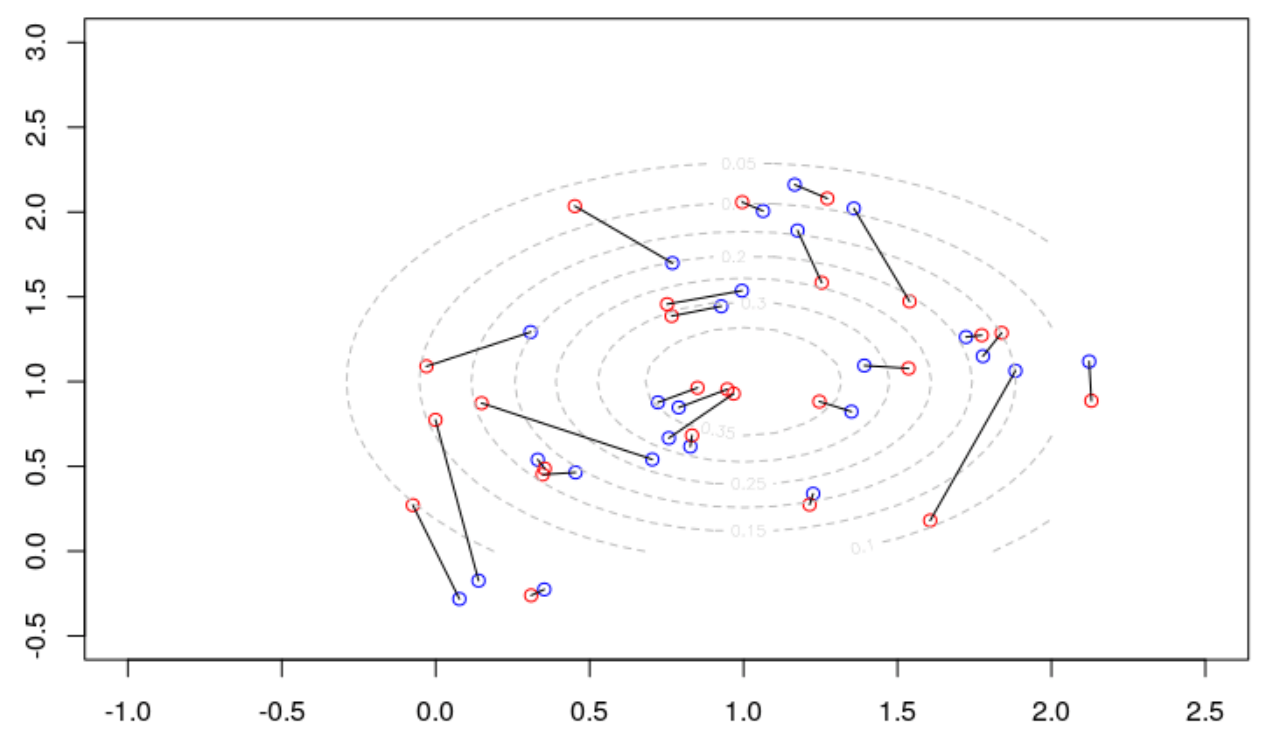Here is the distribution of the total cost, when drawing a thousand samples,

```VC=rep(NA,1000) VA=rep(NA,1000*npoints) for(s in 1:1000){ X1a <- rmnorm(npoints, mean = mu1, Sigma1) X1b <- rmnorm(npoints, mean = mu1, Sigma2) ground_p <- 2 p <- 1 w1 <- rep(1/npoints, npoints) w2 <- rep(1/npoints, npoints) C <- cost_matrix_Lp(t(X1a), t(X1b), ground_p) ab <- transport(w1, w2, costm = C^p, method = "shortsimplex") VC[s]=cout(ab,X1a,X1b) VA[s*npoints-(0:(npoints-1))]=angle(ab,X1a,X1b) } plot(density(VC)```So our cost of 9 obtained initially was not that high. Observe that when drawing from the same distribution, there is now no pattern in the optimal transport

```ag=(VA/pi)*180 ag[ag<0]=ag[ag<0]+360 dag=hist(ag,breaks=seq(0,361,by=1)-.5) polar.plot(dag\$counts,seq(0,360,by=1),main="Test Polar Plot",lwd=3,line.col=4)```Nice isn’t it? I guess I will spend some time next year working on those transport algorithm, since we have great R packages, and hundreds of applications in economics…